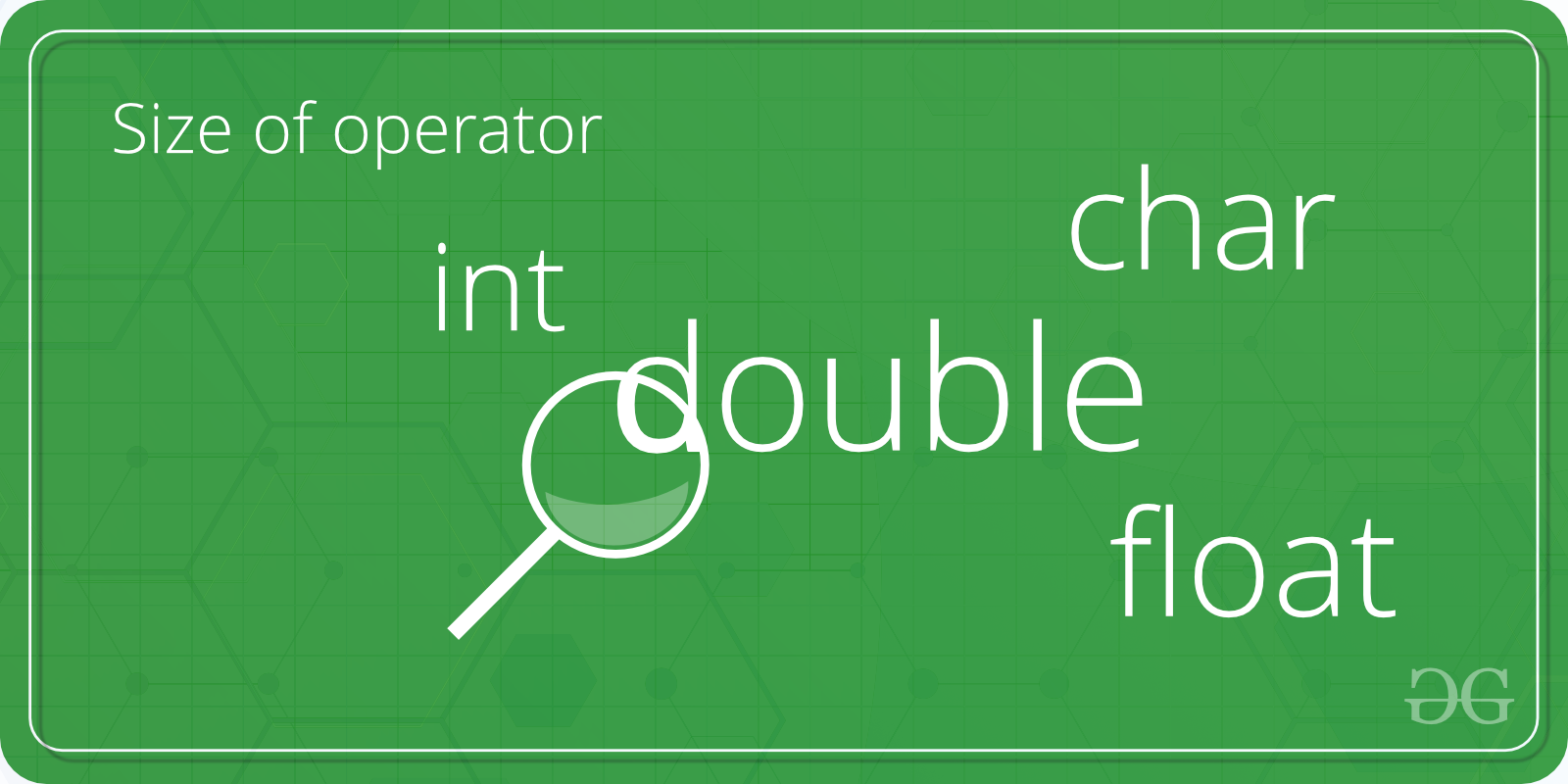# C/C++ program to find the size of int, float, double and char

Given four types of variables, namely int, char, float and double, the task is to write a program in C or C++ to find the size of these four types of variables.Examples:

```Input: int
Output: Size of int = 4

Input: double
Output: Size of double = 8
```

Here is a list of all the data types with its size, range and the access specifiers:

Data Type Memory (bytes) Range Format Specifier
short int 2 -32,768 to 32,767 %hd
unsigned short int 2 0 to 65,535 %hu
unsigned int 4 0 to 4,294,967,295 %u
int 4 -2,147,483,648 to 2,147,483,647 %d
long int 4 -2,147,483,648 to 2,147,483,647 %ld
unsigned long int 4 0 to 4,294,967,295 %lu
long long int 8 -(2^63) to (2^63)-1 %lld
unsigned long long int 8 0 to 18,446,744,073,709,551,615 %llu
signed char 1 -128 to 127 %c
unsigned char 1 0 to 255 %c
float 4 %f
double 8 %lf
long double 12 %Lf

## Recommended: Please try your approach on {IDE} first, before moving on to the solution.

To find the size of the four variables:

1. The four types of variables are defined in integerType, floatType, doubleType and charType.
2. The size of the variables is calculated using the sizeof() operator.

Below is the C and C++ program to find the size of int, char, float and double data types:

## C

 `// C program to find the size of int, char, ` `// float and double data types ` ` `  `#include ` ` `  `int` `main() ` `{ ` `    ``int` `integerType; ` `    ``char` `charType; ` `    ``float` `floatType; ` `    ``double` `doubleType; ` ` `  `    ``// Calculate and Print ` `    ``// the size of integer type ` `    ``printf``(``"Size of int is: %ld\n"``, ` `           ``sizeof``(integerType)); ` ` `  `    ``// Calculate and Print ` `    ``// the size of charType ` `    ``printf``(``"Size of char is: %ld\n"``, ` `           ``sizeof``(charType)); ` ` `  `    ``// Calculate and Print ` `    ``// the size of floatType ` `    ``printf``(``"Size of float is: %ld\n"``, ` `           ``sizeof``(floatType)); ` ` `  `    ``// Calculate and Print ` `    ``// the size of doubleType ` `    ``printf``(``"Size of double is: %ld\n"``, ` `           ``sizeof``(doubleType)); ` ` `  `    ``return` `0; ` `} `

## C++

 `// C++ program to find the size of int, char, ` `// float and double data types ` `#include ` `using` `namespace` `std; ` ` `  `int` `main()  ` `{  ` `    ``int` `integerType;  ` `    ``char` `charType;  ` `    ``float` `floatType;  ` `    ``double` `doubleType;  ` ` `  `    ``// Calculate and Print  ` `    ``// the size of integer type  ` `    ``cout << ``"Size of int is: "` `<<  ` `    ``sizeof``(integerType) <<``"\n"``;  ` ` `  `    ``// Calculate and Print  ` `    ``// the size of doubleType  ` `    ``cout << ``"Size of char is: "` `<<  ` `    ``sizeof``(charType) <<``"\n"``;  ` `     `  `    ``// Calculate and Print  ` `    ``// the size of charType  ` `    ``cout << ``"Size of float is: "` `<<  ` `    ``sizeof``(floatType) <<``"\n"``; ` ` `  `    ``// Calculate and Print  ` `    ``// the size of floatType  ` `    ``cout << ``"Size of double is: "` `<<  ` `    ``sizeof``(doubleType) <<``"\n"``;  ` ` `  `    ``return` `0;  ` `}  `

Output:

```Size of int is: 4
Size of char is: 1
Size of float is: 4
Size of double is: 8
```

Attention reader! Don’t stop learning now. Get hold of all the important DSA concepts with the DSA Self Paced Course at a student-friendly price and become industry ready.

My Personal Notes arrow_drop_upCheck out this Author's contributed articles.

If you like GeeksforGeeks and would like to contribute, you can also write an article using contribute.geeksforgeeks.org or mail your article to contribute@geeksforgeeks.org. See your article appearing on the GeeksforGeeks main page and help other Geeks.

Please Improve this article if you find anything incorrect by clicking on the "Improve Article" button below.

Article Tags :
Practice Tags :

Be the First to upvote.

Please write to us at contribute@geeksforgeeks.org to report any issue with the above content.